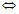# Mechanical set: definition (structure)

## Mechanical set: definition

A mechanical set is defined by:

• a name (and a comment)
• a type of mechanical set

and for the mechanical sets of rotation around one axis and translation along one axis :

• an axis of rotation or translation
• a type of kinematics (= computation mode)
• kinematic characteristics:
• general characteristics
• internal characteristics
• external characteristics
• mechanical stops

## Types of mechanical set

Different types of mechanical sets are presented in the table below.

Type enables the regrouping of the regions corresponding to …
fixed the motionless parts
compressible

the zone of air in which

the moving part is in motion

rotation around one axis

the moving parts

in rotation around an axis

translation along one axis

the moving parts

in translation along an axis

free movement The free motion is designed to take into account complex motions (excluding translation and rotation movement). This motion is described by a list of positions for the mobile part
six degrees of freedom In space, using an orthonormal coordinate system (O,x,y,z), the 6 degrees of freedom are defined as follows:
• Translation along the X axis, Y axis, Z axis
• Rotation around the X axis, Y axis, Z axis

## Types of kinematics

Different types of kinematics (= computation mode) are presented in the table below.

Kinematics (= computation mode) …
of type … enables the realization of a computation …
Multi static

of multi-position type in Magneto Static application

(parametrized study in Magneto Static application)

Imposed speed at imposed speed in Transient Magnetic application
Imposed position at imposed position in Transient Magnetic application

with magneto-mechanical coupling

in Transient Magnetic application

Multiphysics to be able to coupling the motion with another software (like Activate)

## General characteristics

General characteristics gather following information:

• initial conditions (initial speed and initial position)

## Internal characteristics

Internal characteristics gather following information:

• kinematic characteristics relating to the moving part (proper characteristics) (see table below)
Motion Characteristics relating to the moving part
rotating moment of inertia

resistant torque

(formula or 3 coefficients)

translating mass

resistant torque

(formula or 3 coefficients)

## External characteristics

External characteristics gather following information:

• kinematic characteristics relating to the coupled load (external device)

(see table below)

Motion Characteristics relating to the coupled load
rotating moment of inertia

resistant torque

(formula or 3 coefficients)

translating mass

resistant force

(formula or 3 coefficients)

## Mechanical stops

The various mechanical stops are following:

• minimal stop
• maximal stop